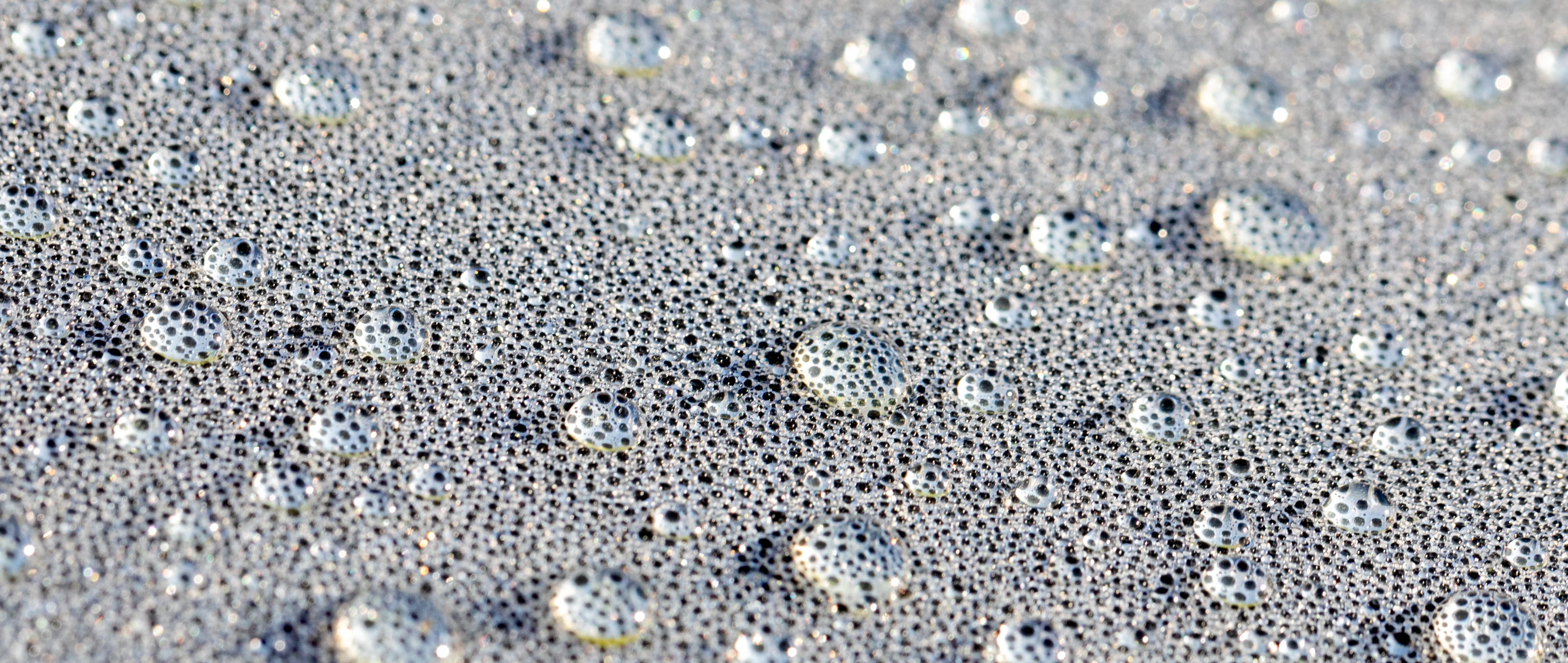Susanna Laurén Jul 5, ’22 > 4 min

# How to utilize contact angles in surface characterization: Roughness corrected contact angle

## Surface Science Blog

We started the series of different contact angles with a static contact angle. The static contact angle offers a fast way of characterizing the wettability of the solid. The static contact angle can, however, be problematic as there is no one static contact angle for any real surfaces. In a typical experiment, you measure the static contact angle on several different spots on the same sample and calculate the average contact angle over the entire area. In some cases, measuring several spots might not be possible, for example, if the area is too small. And even if several measurements are possible, you can end up having a wide spread of contact angle values. One reason for the contact angle variation is surface roughness.

## What is roughness corrected contact angle?

Roughness corrected contact angle, is an angle where the effect of surface roughness is taken away. As a droplet is placed on the surface, it forms a drop whose shape is dependent on the chemical interactions between the surface and the liquid but also the surface roughness. As you measure contact angles, around the same surface, you often see variation between different measurement points. It is impossible to say though, whether the variation is due to surface chemistry or surface roughness variations over.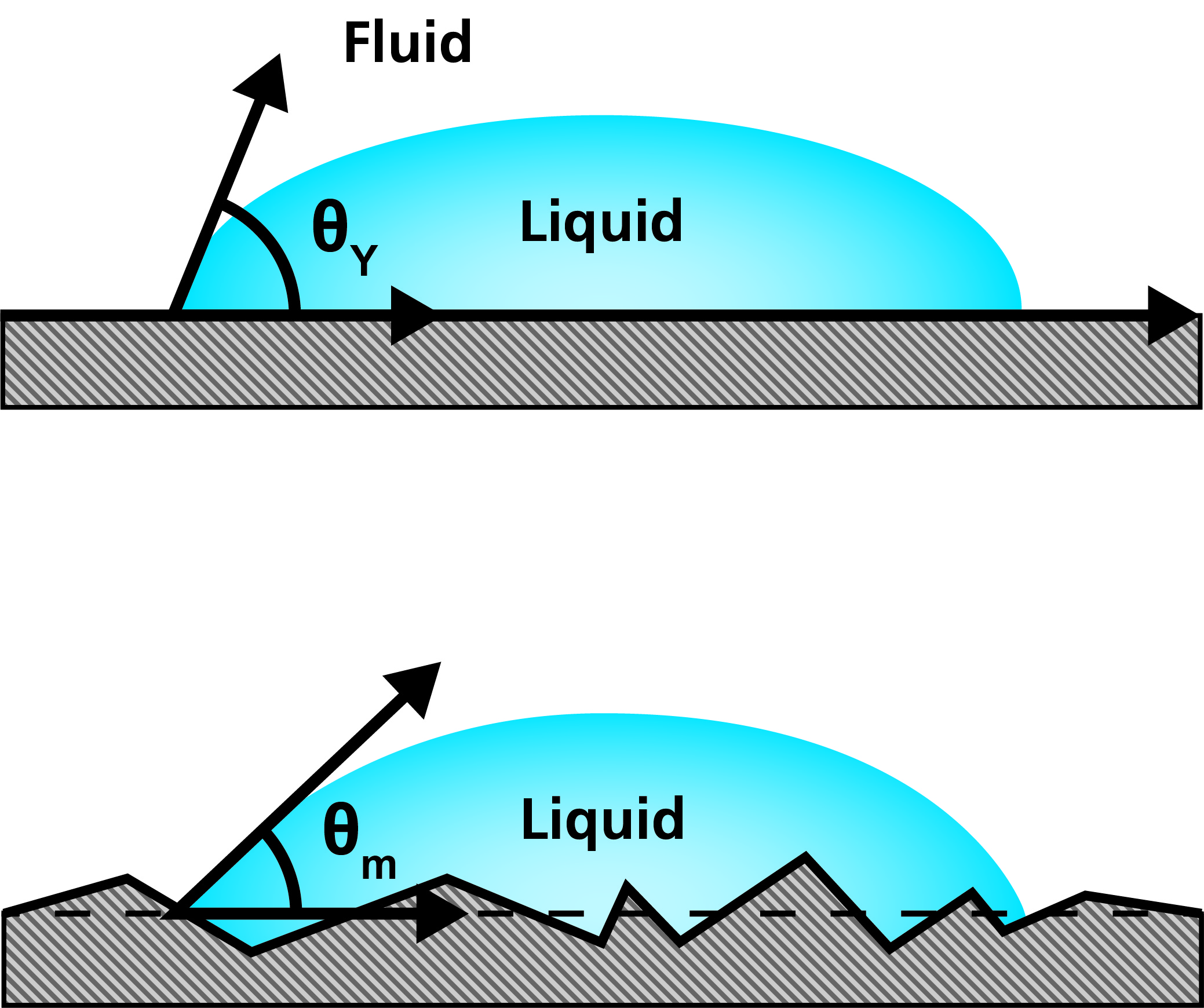The roughness corrected contact angle can be measured by measuring surface roughness on the same spot as the contact angle. The correction is based on the Wenzel equation where the surface roughness factor is used to correlate the measured contact angle to the roughness corrected contact angle.

## Roughness corrected contact angle gives a better estimate of surface free energy

Using roughness corrected contact angles is most suitable for better estimation of surface free energy. Surface free energies are often calculated to be able to compare the surface free energy of the solid to the surface tension of the coating formulation. As you might remember, for the coating formulation to wet the surface, the surface free energy of the solid needs to be higher than the surface tension of the formulation. If the static contact angle is used for surface free energy calculation, the surface roughness can influence the results significantly on rough surfaces. Using roughness corrected contact angles, will take the effect of roughness away from the results. This way the contact angle values used for the calculations are at least somewhat closer to the equilibrium contact angle.

## Problems associated with roughness corrected contact angles

Although roughness corrected contact angles can bring contact angle values closer to the equilibrium contact angle, it does not take into account the chemical heterogeneity of the surface. Also, to be able to use the Wenzel equation for roughness correction, it’s important to make sure that the droplet is at the Wenzel state when on the surface. This means that the drop needs to wet the cavities and holes and no air should be trapped between the surface features.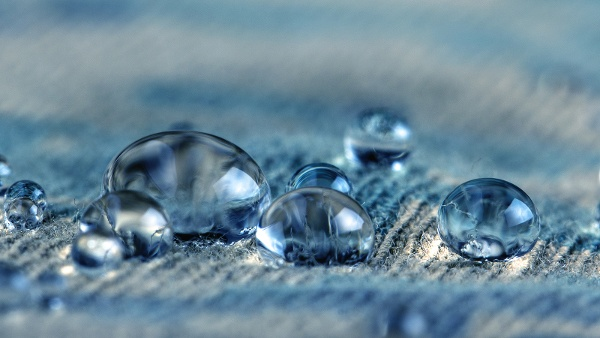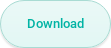## Related products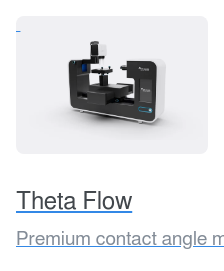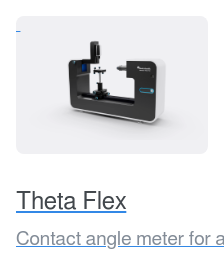## Explore the blog

You have only scratched the surface.